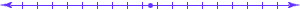## Computer Science 456/656Automata and Formal Languages## What is a Language?

The formal definition is that a language is a set of strings over a given alphabet.
An alphabet is defined to be a finite set whose members are called symbols, but there is no definition of symbol. Thus, anything could be called a symbol.
Examples of languages.
• English, Mandarin Chinese, and other spoken and written languages. We call these "natural languages." We do not study natural languages in this course, yet certain concepts that are in this course, such as context-free grammars, were developed in an effort to analyze natural languages. Serious effort is being made to understand the structure of natural languages, and anyone doing serious research in that area must have extensive knowledge of the subject matter of this course.
• Formal mathematical languages, such as decimal numeration that you learned as a small child, binary numeration, the language of algebraic expressions you learned in school, or the language of formal logic you learned in PHI 109.
• Pascal, C++, and other programming languages. These languages incorporate the complexity of formal mathematical languages and much more. I will assume that you are familiar with at least one programming language and have written computer programs.
• The genetic code. All living creatures, as far as we know, utilize a language whose symbols are molecular groups, and which is written in DNA or RNA.
• 0-1 problems. This is probably a surprise use of the word "language" to most of you. Most people usually think that a language must always be used for communication, as are all of the above examples. But there is no requirement that a language be useful for anything.
A 0-1 problem is a usually thought of as a class of questions where the answer is always either "no" or "yes." For example, we would usually think of the "Addition Problem" as all questions where we are asked if the sum of two numbers is equal to a third number, such as, "Is it true that 2+3=5?" or "Is it true that 2+2=6?" Such individual questions would be called "instances" of the problem.
To think of a given 0-1 problem as a language, we must first encode all instances using some alphabet, and then define the language to be all such encodings where the answer is "yes." For example, all instances of the addition problem can be encoded using 12 symbols, the digits, the plus sign, and the equals sign, and the language Ladd can be defined to be all those strings where the equation is true. For example, the strings "2+3=5" and "439+21=460" are members of Ladd, while the strings "2+2=6" and "+34=+" are not, in the first case, because it is the encoding of an instance where the answer is "no," and in the second case because it is not the encoding of any instance.
The box at the top of page 33 of Hopcroft, Motwani, and Ullman discusses this important use of languages.